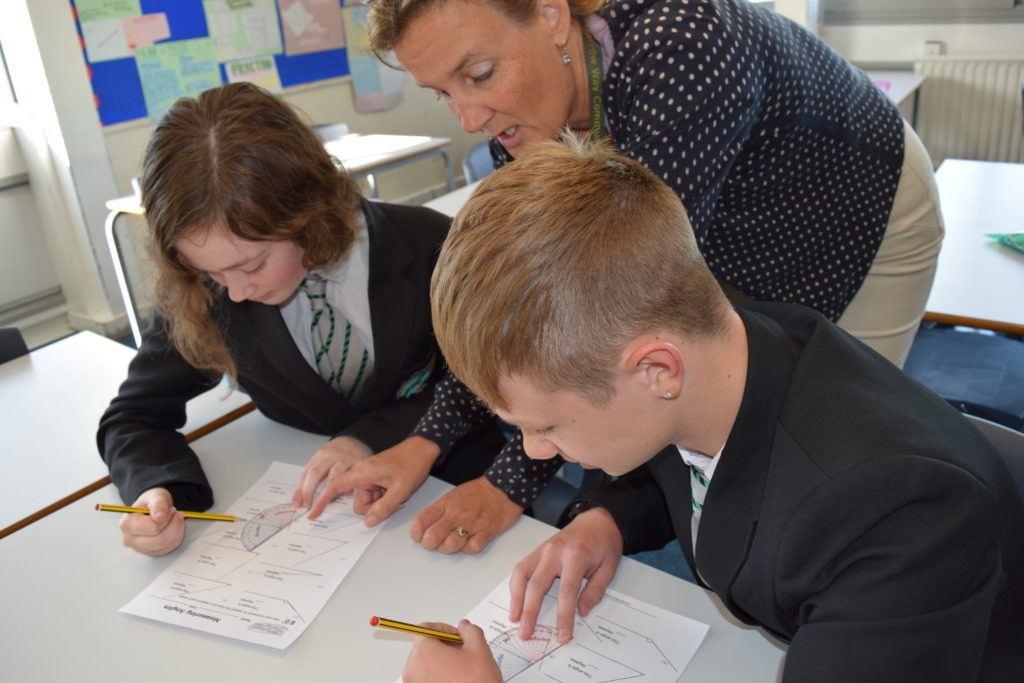## Welcome to the Mathematics Department

As a Mathematics and Computing Specialist School, we are passionate in the belief that every student should be able to access all aspects of Mathematics in a way that will benefit their choices in life.

Understanding and using numeracy is key to the development of student’s knowledge and success. All students are given the opportunity to extend their skills regardless of ability. Lessons are taught using a variety of methods including written, practical and investigative exercises, whilst making full use of computer software, where appropriate, in order to provide for all learning styles. In addition to formal lessons, students are able to gain experience from taking part in extra-curricular activities.

### Curriculum Content

#### Year 7

• Basic Number
• Basic Algebra
• Tables, Charts and Diagrams
• Shape & Angles
• Problem Solving Weeks
• Coordinates and Graphs
• Basic Constructions
• Pythagoras Theorem
• Basic Averages
• Basic Probability

#### Year 8

• Number: Percentages, Sequences, Highest common Factor, Lowest Common Multiple, Ratio and compound measures, Basic Standard Form, Mixed numbers
• Algebra: Brackets, Equations, incl. Basic Simultaneous Equations, Graphs
• Statistics: Scatter Graphs, Probability, Averages
• Shape & Space: Angles in Polygons, Volume of 3D Shapes, Circles, Transformations, Loci and Bearings, Basic Congruence and Similarity
• Problem Solving: Developing skills in Estimation, Sequences and Lowest Common Multiple, Ratio and Compound Measures

#### Year 9

In Year 9, students consolidate their learning and knowledge acquired in the previous two years. They form a strong foundation to succeed at GCSE level. All aspects of the KS3 National Strategy for Mathematics are completed. At the same time, students develop their mathematics skills further in preparation for a smooth transition to KS4. The following aspects of the curriculum are covered:

• Basic number
• Basic algebra
• Averages and tables
• Angles
• Data, Charts and Diagrams
• Pythagoras’ Theorem
• Transformations of shapes
• Basic probability
• Sequences
• Ratio and compound measures
• Linear graphs
• Problem solving and reasoning#### Key Stage 4

At Key Stage 4 students study Edexcel (9-1) GCSE Mathematics.
This Edexcel GCSE in Mathematics qualification requires students to:

• Develop deeper knowledge, skills and understanding of mathematical methods and concepts and problem solving skills in the following areas:
• Number
• Algebra
• Geometry
• Measures
• Rates of change
• Statistics
• Probability
• Use their knowledge and understanding to make connections between mathematical concepts
• Apply the functional elements of mathematics in everyday and real-life situations
• Solve problems in familiar and unfamiliar context using their mathematical skills

The examination is made up of one non-calculator paper and two calculator papers each worth one third of the final grade.

It is our intention that all students leave Harrow Way Community School having had a positive mathematical experience as well as achieving their full potential in the subject.